# Are we Golden?

Anyone who gets into design soon enough comes across the Golden Mean, represented by the symbol ‘Φ’, and expressed as a number value approximately equal to 1.6180339887….This number is tremendously important and worth knowing about, and though it may be a bit pedantic on my part, I would like to try and explain it as best I can as a carpenter/designer, for the benefit of those who would rather do anything than look at math books. After a walk into the forest to give – hopefully, a clear explanation of what phi is in this post below, then later on we can look at why you might want to make use of phi, and even why nature is organized around phi.

This number, termed phi (the ‘i’ pronounced as in ‘ice’) can only be expressed as an approximation because it is irrational. ‘Irrational’ doesn’t mean ‘illogical/crazy’, but rather that the number cannot be expressed with a ratio, numerator over denominator, like 1/2, 55/64, and so forth. This fact in itself makes phi quite an mysterious number indeed, and it is in good company with other irrational numbers like √2 and √3. To put it concretely, if you had a room with a floor space measuring 1.0 on one side, and 1.618…, Φ, on the other, there is no size of square parquet tile that can be used to tile the floor area evenly. It doesn’t matter how small you make the tile. That’s pretty weird, at least it is to me.

Phi is also described as the ‘Golden Mean’, ‘Golden Section’, or the ‘Golden Ratio’. The term ‘mean‘ is as in ‘average amount or value, or middle-state or position‘, and can be expressed in the following graphic:The ratio of the whole line segment ‘C’ to the large segment ‘A’, is the same as the ratio of the large segment ‘A’ to the small segment ‘B’. In other words, ‘C’ is to ‘A’ as ‘A’ is to ‘B’.
To the whole line segment ‘A’ I have projected down to a black dot indicating the location of the Golden Mean point. The relations between the lines ‘A’, ‘B’, and ‘C’ above can only occur when ‘C’ is 1.6180339… times greater than ‘A’, and line A length is 1.6180339… times greater than B. The ancient Greeks called this “dividing the line by it’s mean and extreme ratio”.

That’s all very nice, but suppose you didn’t have a calculator and hadn’t memorized that value of Φ – how would you find the value of the Golden Mean – that is, find a line like ‘A’ above, which is 1.6180339887… longer than ‘B’? It turns out this is fairly simple to do, so long as you have a compass and straightedge handy. Now, no cheating by using graph paper. First you draw a straight line, ‘A’, at your given starting line segment length, and then use your compass to construct a square from it – that is, all 4 sides are of equal length and corners are 90˚. Once you have the square drawn, again take your compass and use it to divide the square in half, as illustrated:Then take your straightedge and draw a diagonal, constructing a right angled-triangle, with one side equal to ‘A’, and the other equal to A/2. Take the length of this diagonal on the compass, and swing the arc down to the floor, as shown:Project the various points indicated down to produce your line segment, and voila! you have produced the Golden Mean length, depicted in red: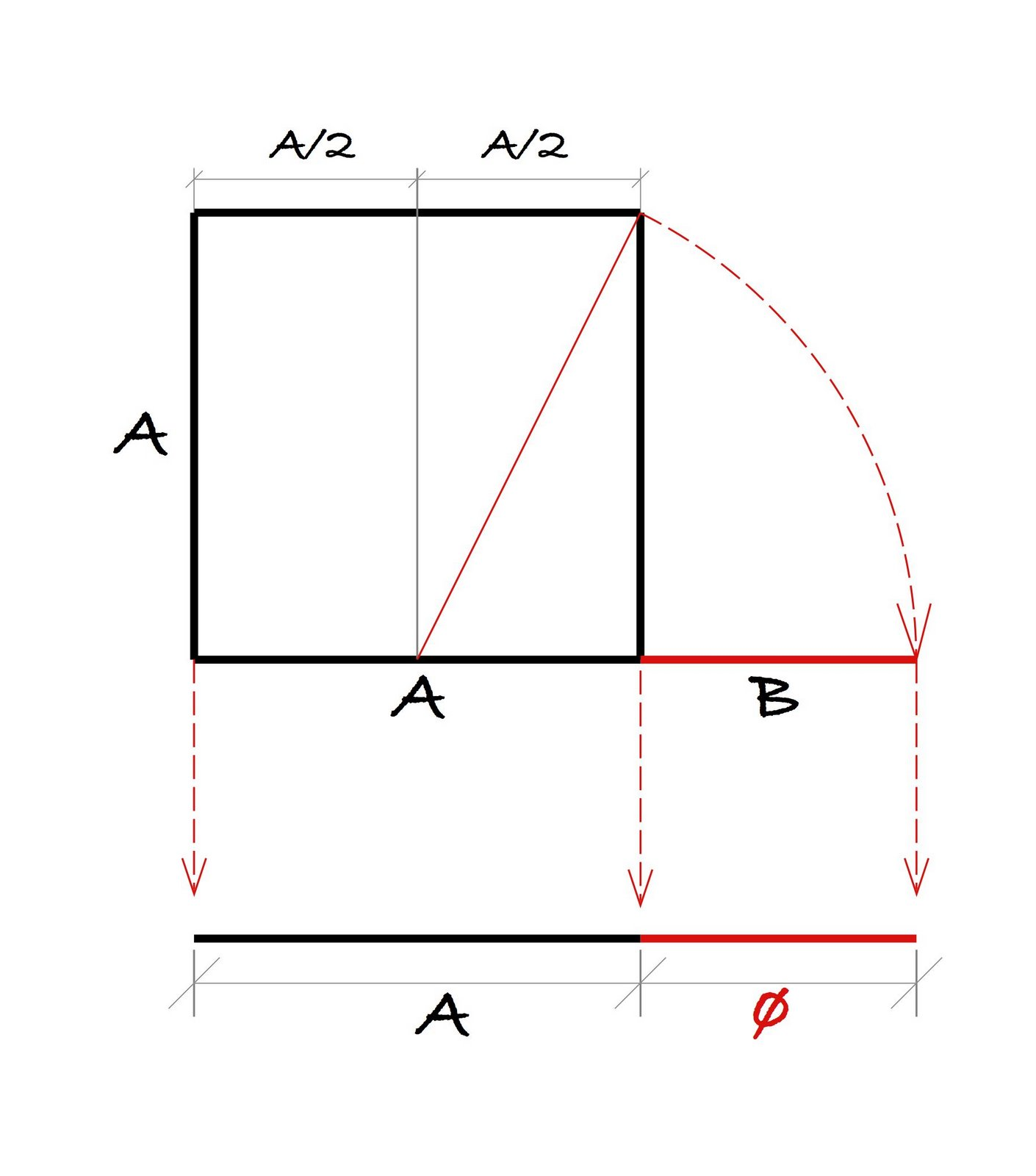Now, the above method might not work for you if you had to start with a particular length of line upon which to find a portion of that line equal to the Golden Mean. In other words, in the the initial explanation above giving the lines ‘A’, ‘B’, and ‘C’, let’s say you had an existing line length segment of ‘C’ and wanted to find the portion of it equal in length to segment ‘A’ (that is, segment ‘C’ divided by the golden mean value of 1.6180339…), what then?

It turns out that rocket science is required to find it – just kidding! It’s a fairly similar sort of procedure to the aforementioned one. This time, start with your line ‘C’. Use your compass to construct a perpendicular at the end of the line. Now the real building can begin!

Okay, I realize this particular drawing procedure might be a little fuzzy for some – in fact they may well have omitted the technique in elementary school, so it seems worth taking the time to look at. The method of making a perpendicular at the end of a line is definitely a useful drawing technique for any carpenter or woodworker to have at their disposal, as it comes up frequently, and we might not always have an accurate square to use, or the size of perpendicular might be quite long and using a square therefore inaccurate.

To construct an ‘end-line perpendicular’ then, take your given line segment and set your compass to about one-half the length of the line. Place the compass at one end of the line, at ‘A’, and scribe an arc, as shown: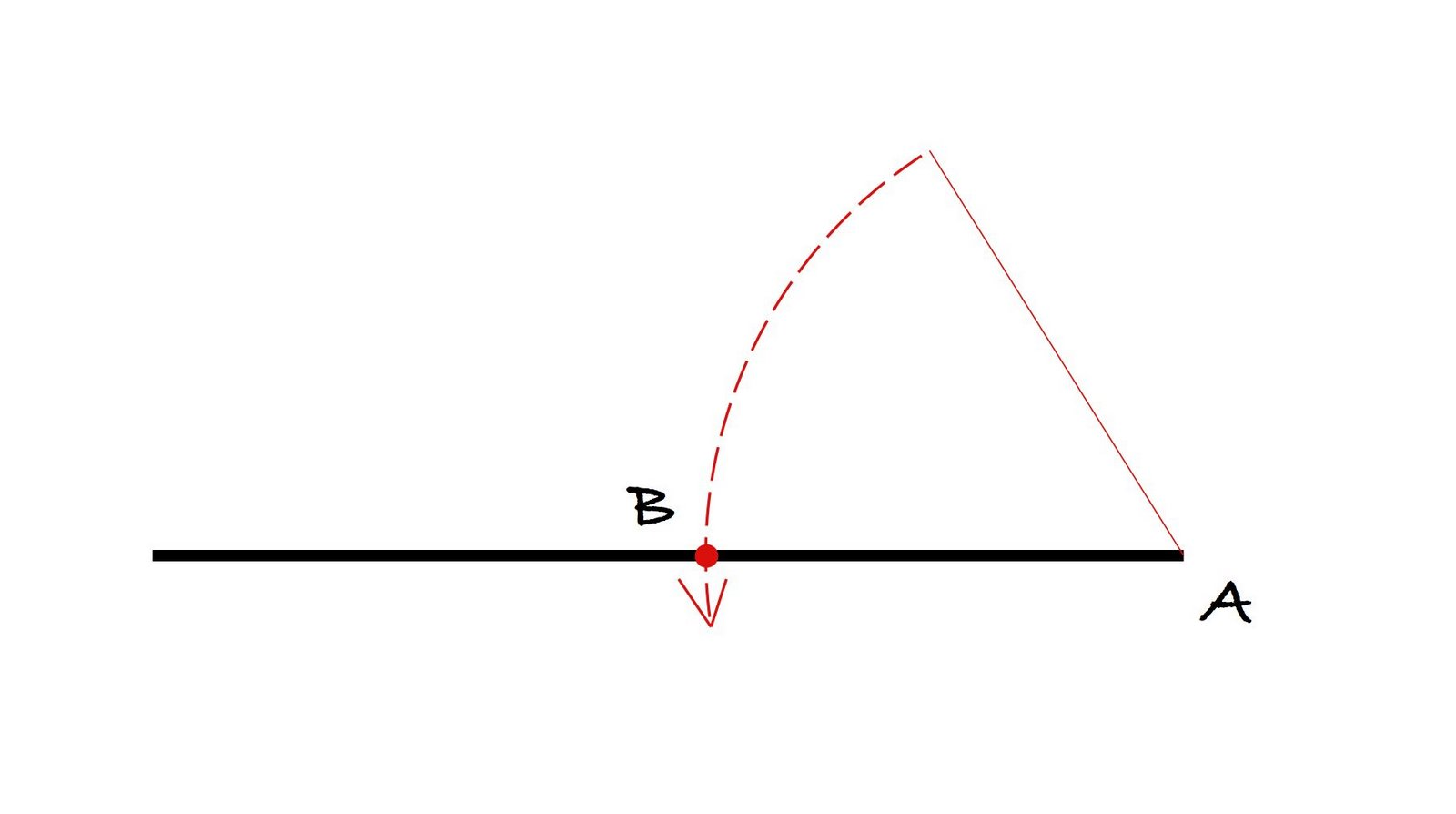This creates point ‘B’ at the intersection of the arc and the line segment. Take the compass, without changing the setting, and scribe another arc, using point ‘B’ as the center, vis: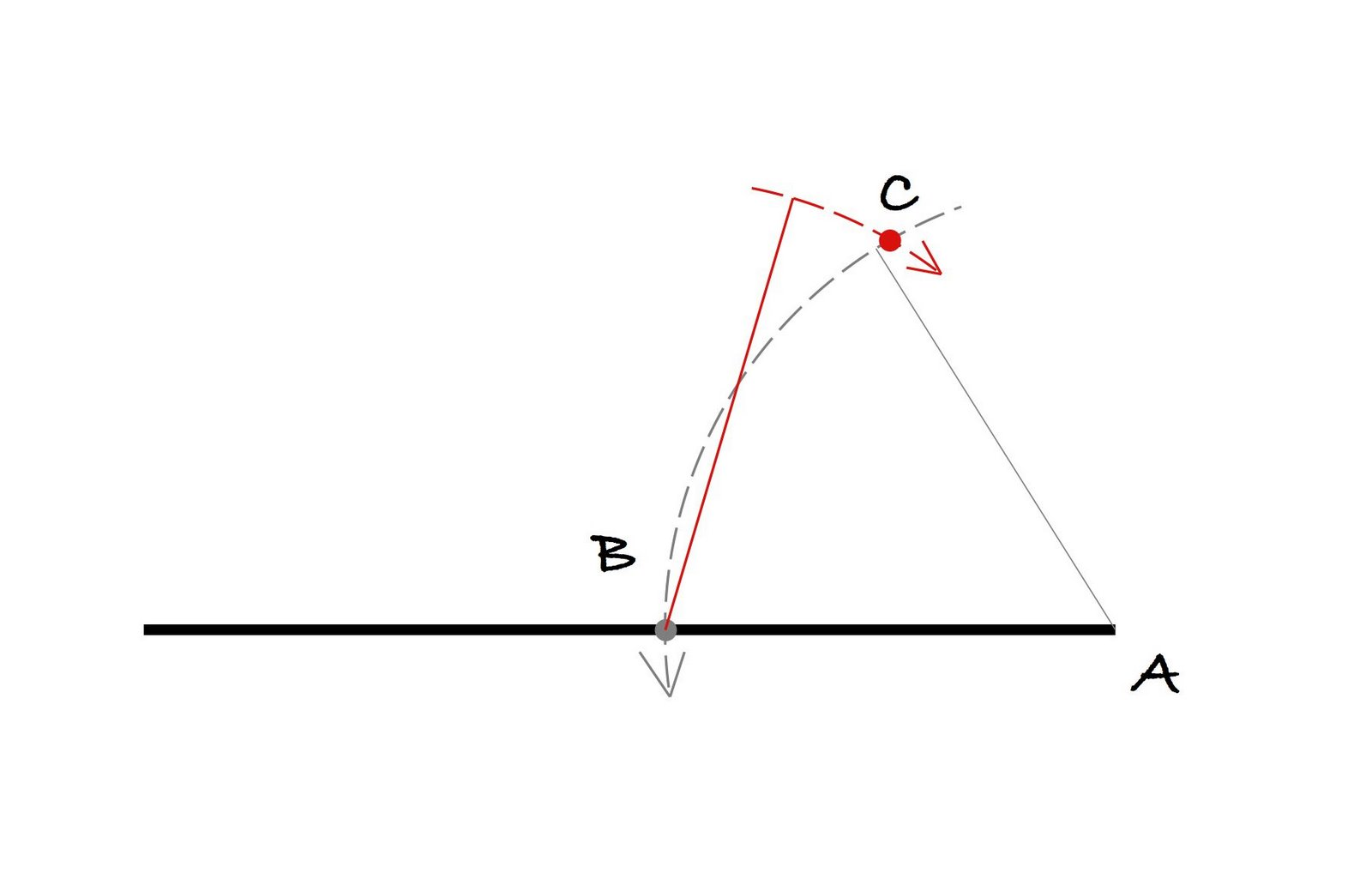This gives us point C. Triangle A-B-C, if you notice, has all three sides of the same length, termed equilateral ( meaning “equal sides”), and therefore internal angles are all equal. Since there are 180˚ in a triangle, each equal angle here is therefore 60˚. If you need to draw a 60˚ angle sometime, all it takes is two swings of a compass.

Take your straightedge and connect points ‘B’ and ‘C’, projecting off and up to the right: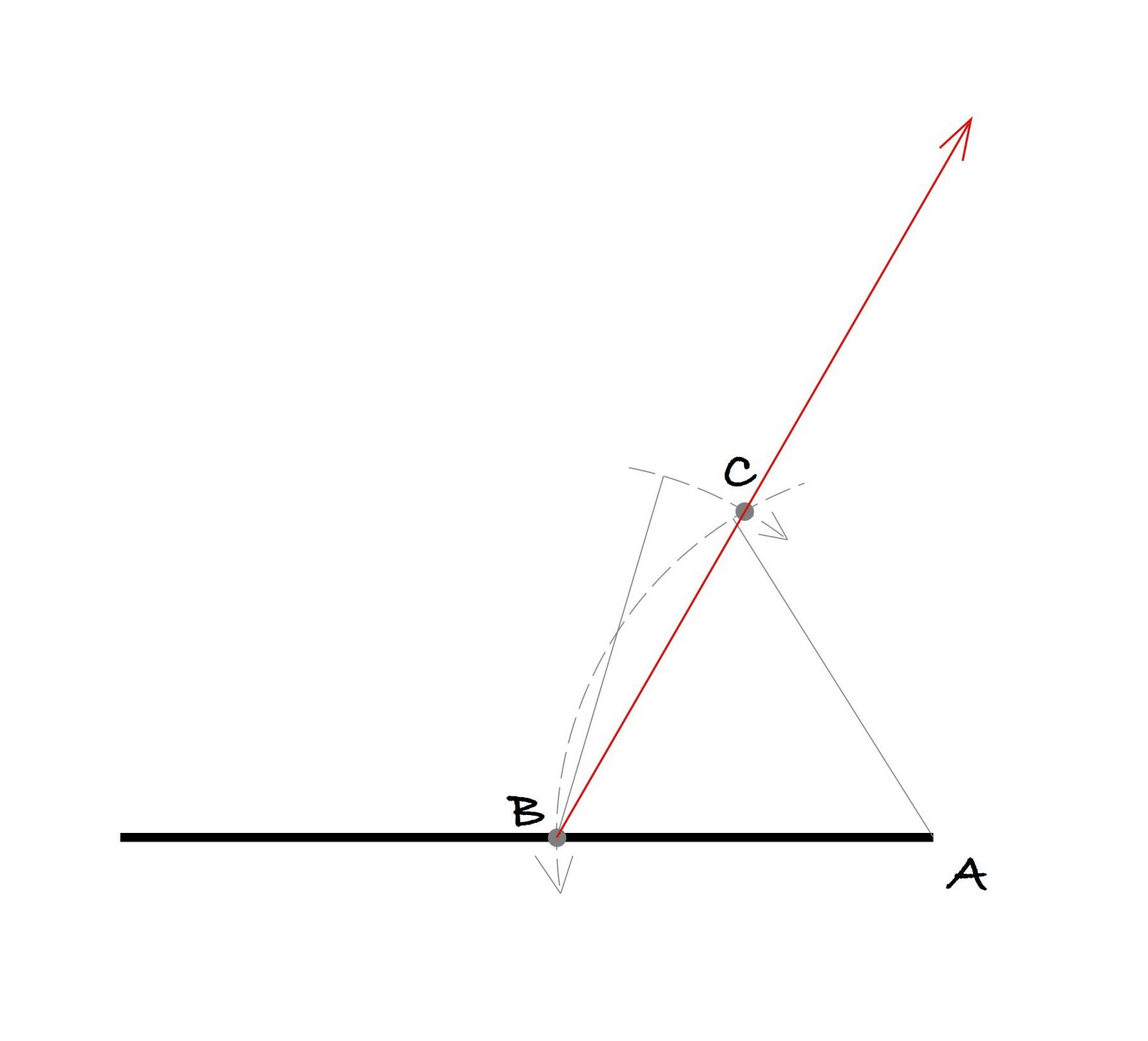Now take your compass, same setting, and place the point at ‘C’, and mark another arc as shown, giving point ‘D’: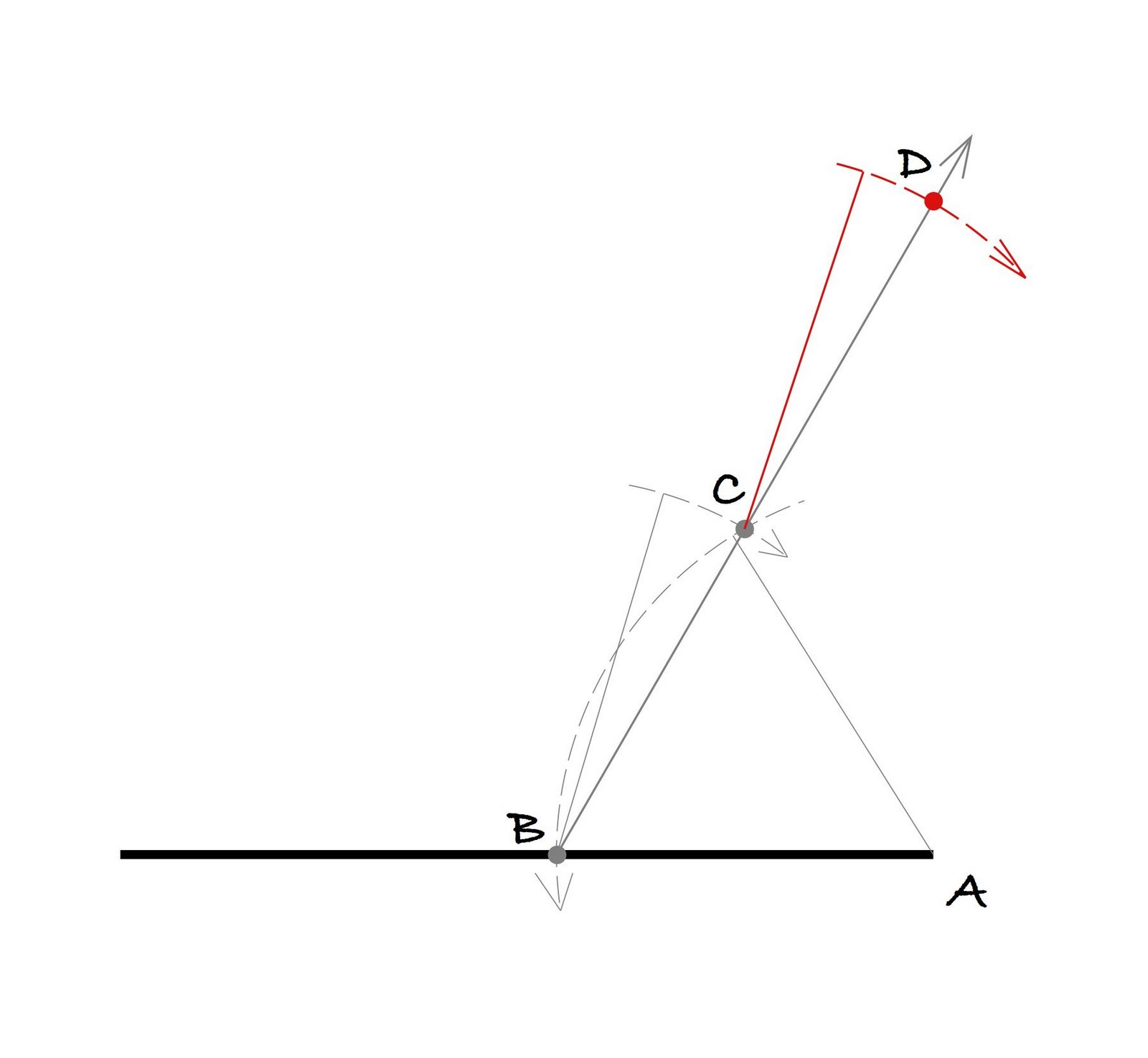You can see then that the length from B~D is twice that of A~B. Almost there. Connect points ‘D’ and ‘A’ with a straight line, and there you have your perpendicular: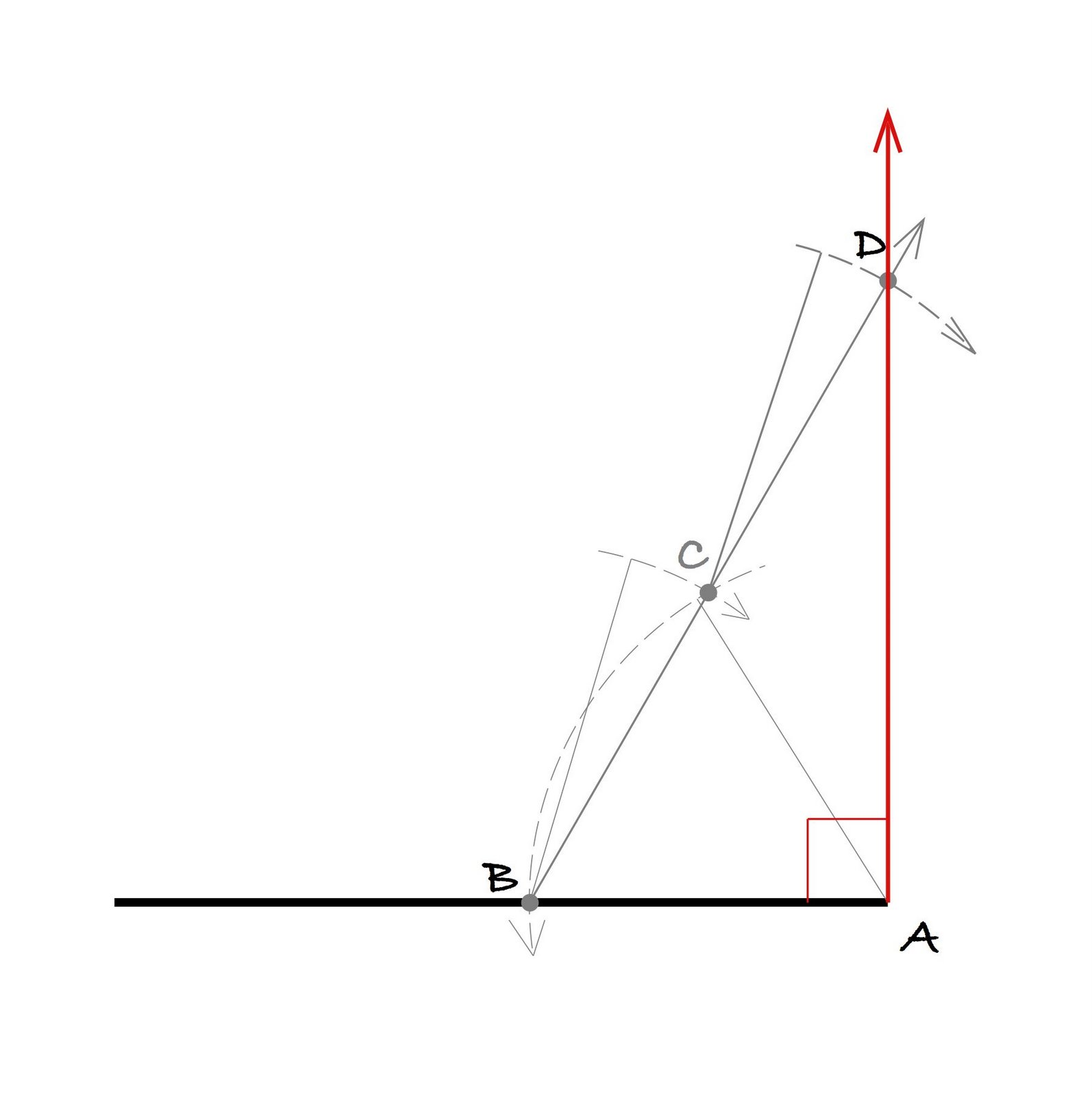Next step is to divide your initial line segment into two equal pieces, in the same manner as for the method deriving phi from the square earlier:Connect the interesting arcs, top and bottom, with a perpendicular to your line segment that divided the line equally in two, at point ‘F’. Distance A~F and E~F are equal.

Now reset the compass to the 1/2 segment distance A~F and swing an arc through the perpendicular established at the end of the line segment: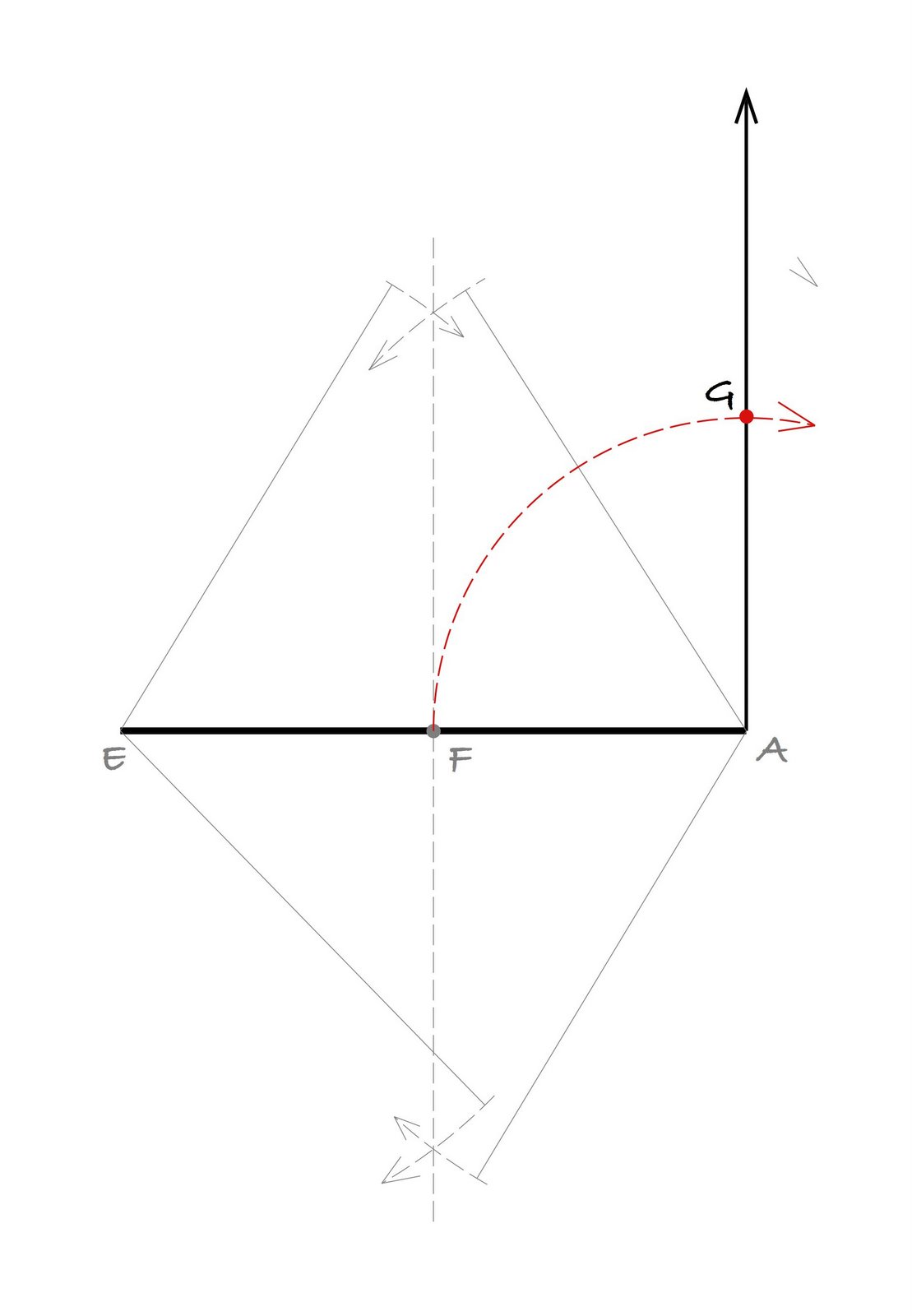This gives point ‘G’. Connect ‘E’ to ‘G’ and we make a right-angled triangle:This is a triangle with a rise, A~G, equal to half, 0.5, of the run. The run A~B therefore is 1.0 units in length.

Keeping your compass at the same 1/2 segment length you just used, swing an arc from ‘G’ through the hypotenuse of the triangle:Now take the compass, fixing the point at the opposite end of the triangle, and fixing the length to the intersection point just established. swing this arc down to the line segment as shown:Where this line crosses the line segment at the point shown, then projected down square to the line segment, you will see that we have now divided the original line segment into “extreme and mean proportion”. Tah-dah! Have a beer.

Let’s see what that value is using a calculator. Rise is 0.5, run is 1.0. Hypotenuse is found using Pythagorean method: C-squared=A-squared + B-squared. To find the value of ‘C’ alone, we take the square root (√) of both sides:

hypotenuse= √(run-squared + rise-squared)

√(1.0-squared + 0.5-squared)

= √(1.25)

Since the length of the hypotenuse is 1.1180339887…, and we swung the length of the rise, 0.5, up to intersect it, we find the length of remainder of the hypotenuse (that second arc) then to be:

1.118033887… – 0.5 = 0.6180339887….

hmmm.

Here’s that same drawing with this information summarized: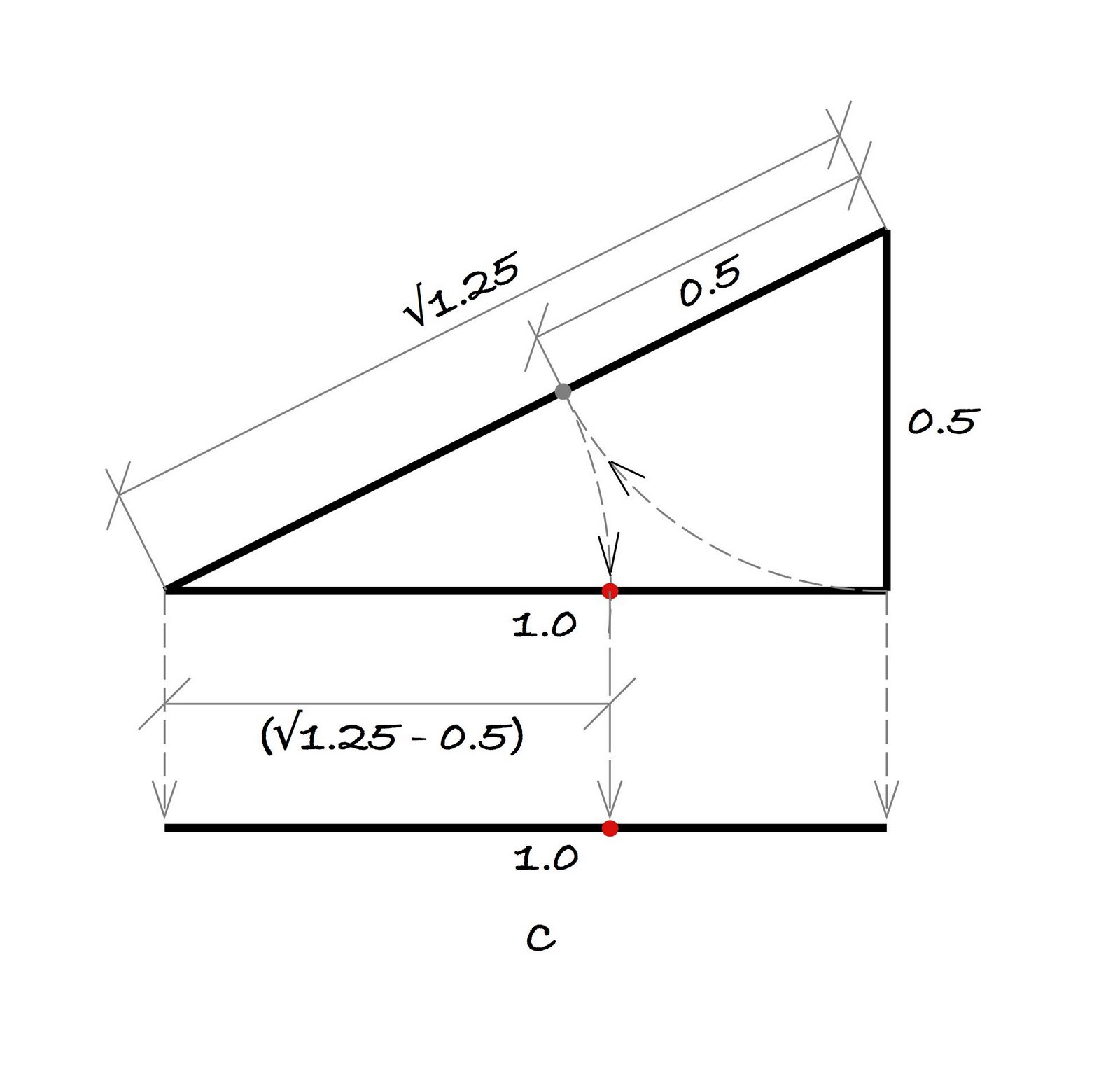You might recall earlier, from the beginning of time when I started this post, that I said the value of phi, Φ, is 1.6180339887, yet in the above example we seem to find a number a little different, yet curiously with the same remainder to the right of the decimal. How’s that? Well, curiously, if you take the length of our segment ‘C’ and divide it by 1.6180339887.., you get the value of 0.6180339887..!!

Phi is a very curious number, and the only one I’m aware of with this property. Also, of course, Φ-1=0.6180339….

Another way of finding the golden ratio from the same triangle is found as follows, this time we’re taking the run to be 2.0 and the rise to be 1.0. By Pythagorus, the hypotenuse therefore measures √5, or 2.236067978…. Here, instead of swinging the rise to divide the hypotenuse into two portions, we swing it the other way to add to the hypotenuse: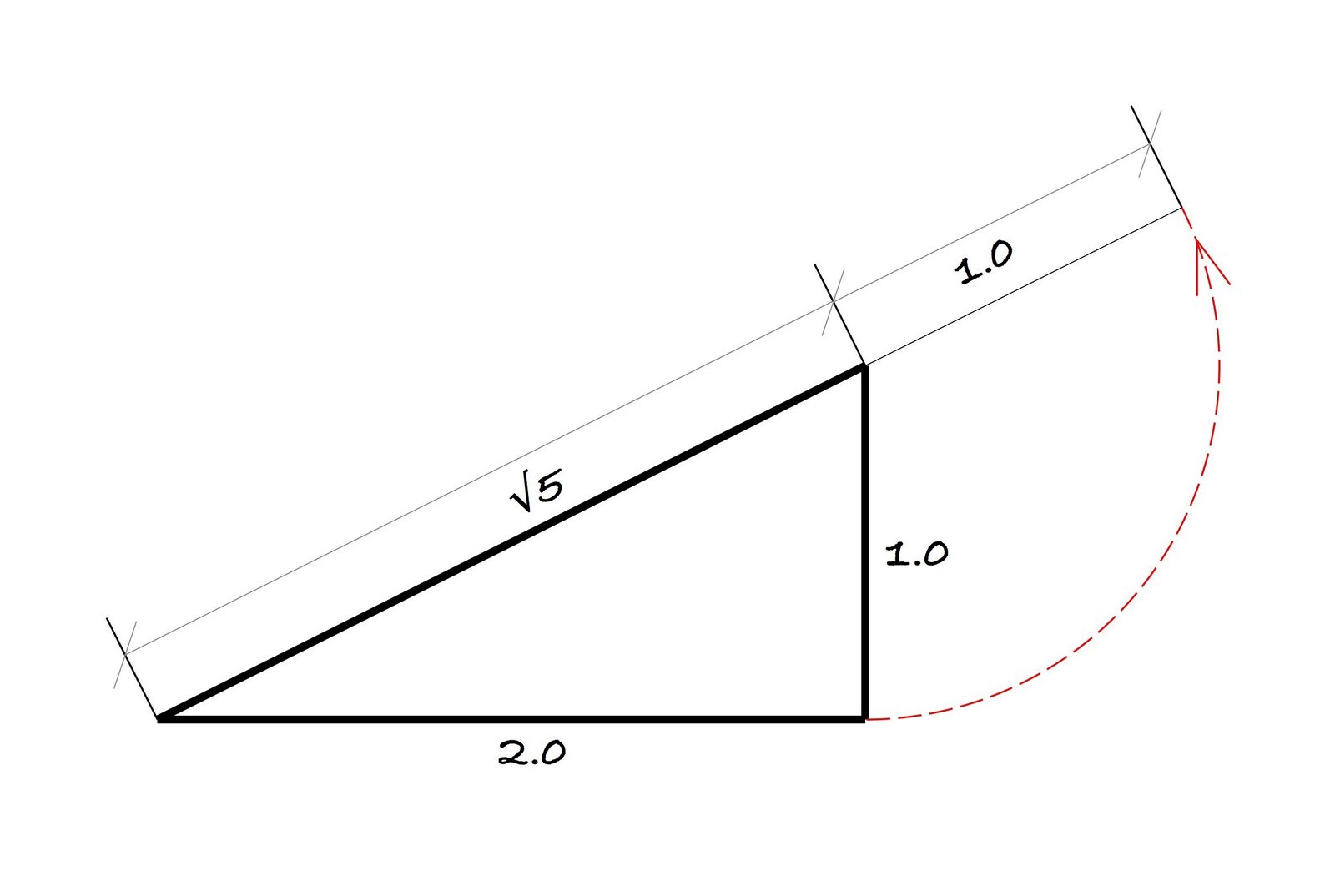Now we take the ‘extended hypotenuse length’ and divide it in half using the compass:It should be apparent why by looking at the drawing too. As you can see, the division of the extended hypotenuse is given by the formula (√5 +1)/2. If you work out that formula on your calculator, you will see it produces the value of 1.6180339887, or phi. And the remainder of the extended hypotenuse, on the upper side of the dividing line cutting it in two, gives the other value of phi, as shown:As in the first example with lines, the segments of the hypotenuse relate by golden ratio; B~E is to B~D, as B~D is to A~E.

By the way, in order to avoid confusion between the two values for phi, there is what you might call an ‘upper case’ phi, a multiplier of 1.6180339887.., denoted by the symbol Φ, and there is a ‘lower case‘ phi, 0.6180339887… which is denoted by the symbol φ

Almost done. Here’s the weird thing now. If you say “divide a number by 2“, one could also say, “multiply by 0.5“, since 1/2=0.5. That is, 10/2= 10 x 0.5.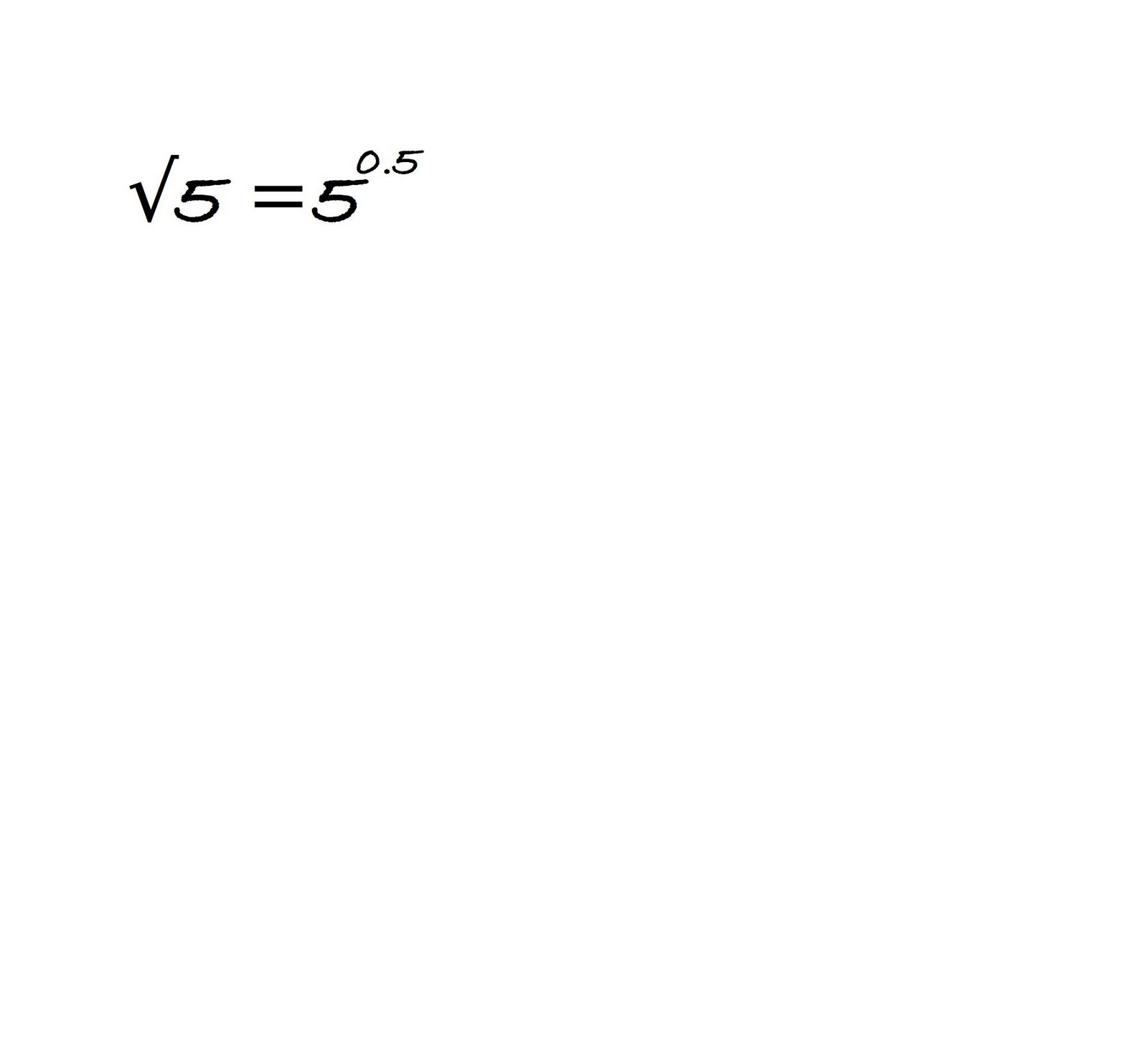Also, one can say, “take the square root of a number“, or one can say “raise it to the power of 0.5“. I can’t easily type in exponents on the computer for some goofy reason (and I thought tech geeks invented these machines ?), so we use the symbol ‘ˆ’, to mean ‘raised to the power of ___‘.

Looking back at the geometrical derivations we explored, and the way we ‘crunched’ the numbers, so to speak, to find that phi was given by the formula (√5 +1)/2.

That could be written differently as (√5/2)+(1/2)

Another way of writing that then is: 5ˆ0.5 x 0.5 +0.5 (!)

Lots of 5’s in that formula! There is a connection between the number 5 and the golden ratio, and that’s what I wanted to get into in part II of this series. Excuse the length here folks – where did the day go?

error: Content is protected !!